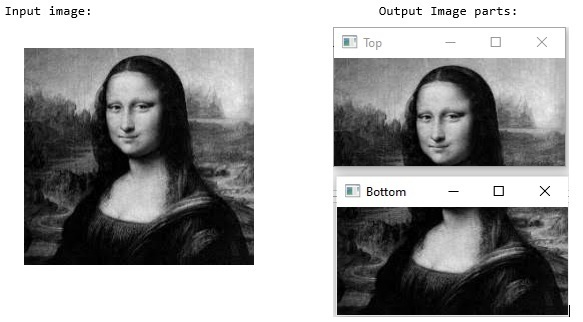# Dividing Images Into Equal Parts Using OpenCV Python

The Python OpenCV library enables us to utilize a variety of image-processing tools, like image classification, face/object detection, tracking, and more.

In this article, we will use python list slicing or numpy array slicing techniques to divide an image into equal parts, since OpenCV-python uses the Numpy array to store the image data/pixel values.

## Input Output Scenarios

Assuming we have an input image and in the output, we will see the equally divide parts of the given image.### Approach

We will follow the below steps to divide the images into equal parts.

• Extract the image dimensions and store them in a variable.

• Divide the image array using the python slicing technique.

• Finally save the segregated parts.

### Example

In this example, we will horizontally divide the input image “cat.jpg” into 2 parts.

import cv2

height, width, channels = image.shape
half_height = height//2

top_section = image[:half_height, :]
bottom_section = image[half_height:, :]

cv2.imshow('Top', top_section)
cv2.imshow('Bottom', bottom_section)

cv2.waitKey(0)


### Input Image### Output Images### Example

In this example, we will divide the input image “logo.png” into 4 equal parts.

import cv2
import numpy as np
def divide_img_blocks(img, n_blocks=(2,2)):
horizontal = np.array_split(img, n_blocks)
splitted_img = [np.array_split(block, n_blocks, axis=1) for block in horizontal]
return np.asarray(splitted_img, dtype=np.ndarray).reshape(n_blocks)

for i in range(result.shape):
for j in range(result.shape):
cv2.imwrite(f"Output Images/my_block_{i}_{j}.jpg", result[i,j])


### Input Image### Output Images### Example

In this approach, we will divide the input image “Lenna.png” into 9 equal parts.

import cv2,time

img2 = img

height, width, channels = img.shape
# Number of pieces Horizontally
W_SIZE  = 3
# Number of pieces Vertically to each Horizontal
H_SIZE = 3

for ih in range(H_SIZE ):
for iw in range(W_SIZE ):

x = width/W_SIZE * iw
y = height/H_SIZE * ih
h = (height / H_SIZE)
w = (width / W_SIZE )
print(x,y,h,w)
img = img[int(y):int(y+h), int(x):int(x+w)]
NAME = str(time.time())
cv2.imwrite("Output Images/" + str(ih)+str(iw) +  ".png",img)
img = img2


### Output

0.0 0.0 124.0 223.0
223.0 0.0 124.0 223.0
446.0 0.0 124.0 223.0
0.0 124.0 124.0 223.0
223.0 124.0 124.0 223.0
446.0 124.0 124.0 223.0
0.0 248.0 124.0 223.0
223.0 248.0 124.0 223.0
446.0 248.0 124.0 223.0


### Input Image### Output ImagesThe above example, will divide the image first into 3 Horizontal pieces then for each of that 3 pieces it will crop another 3 images Leaving a total of 9 parts. Change the W_SIZE and H_SIZE value to adjust how many equal parts we need to divide the image.

In all the above examples, we have successfully divided the input images into equal parts.

Updated on: 30-May-2023

1K+ Views